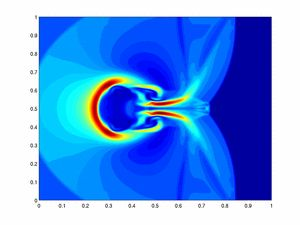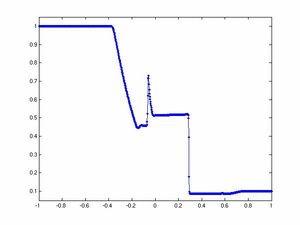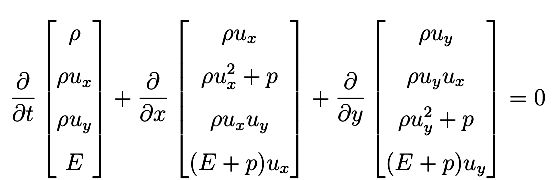## AMSC 612, Fall 2015

### Course Information

 Place 4122 CSIC Bldg. #406 (unless otherwise stated) Time Tuesdays & Thursdays, 14-15:15pm Tuesday and Thursday Nov. 3-5 meetings, 14-15:15pm: class at CSIC 4141 Additional meetings: Wednesdays 10-12pm on Sep. 30, Nov. 18 and Dec. 2 Instructor Professor Eitan Tadmor Contact tel.: x5-0648   Email: eitan Tadmor Office Hours By appointment Midterm Thursday, October 15 4122 CSIC Bldg. #406 Final Due: Fri. Dec 11, 2pm 4145 CSIC Bldg. #406 Grading 50% Homework + midterm, 50% Final

### Course Description

Time-dependent Partial Differential Equations (PDEs) of hyperbolic and parabolic type. Initial and initial-boundary value problems. Finite difference and spectral methods for time-dependent problems. The linear theory: accuracy, stability and convergence. Nonlinear problems: shock discontinuities, viscosity and entropy. Finite volume and discontinuous Galerkin numerical methods.#### Introduction

• Examples of nonlinear conservation laws: Euler and Navier-Stokes equtions, the shallow-water eqs.
PDEs from image processing (examples (i) and (ii)), geometrical optics, incompressible Euler eqs, nonlinear models for traffic flow,...
• Fron nonlinear to constant-coeffiecinets: linearization, method of characteristics
Assignment #1 [ pdf file ] with selected answers [ pdf file ]

#### Initial Value Problems

• Initial value problems of hyperbolic type
• The wave equation - the energy method and Fourier analysis
• Weak and strong hyperbolicity -- systems with constant coefficients
• Hyperbolic systems with variable coefficients
Lecture notes on the one-dimensional wave equation (J. Balbas)

• Initial value problems of parabolic type
• The heat equation -- Fourier analysis and the energy method
• Parabolic systems
• Well-posed problems
Lecture notes: Time dependent problems [ pdf file ]

Lecture notes: Matrices: Eigenvalues, Norms and Powers [ pdf file ]

#### Finite Difference Approximations for Initial Value Problems

• Preliminaries
• Discretization. grid functions, their Fourier representation, divided differences.
• Upwind and centered Euler's schemes: accuracy, stability, CFL and convergence
Assignment #2 [ pdf file ] with selected answers [ pdf file ]

Related material:  [ CFL paper 1928 ]

• Canonical examples of finite difference schemes
• Lax-Friedrichs schemes: numerical dissipativity
• Lax-Wendroff schemes: second- (and higher-) order accuracy
• Leap-Frog scheme: the unitary case
• Crank-Nicolson scheme: implicit schemes
• Forward Euler for the heat equation
Assignment #3 [ pdf file ] with selected answers [ pdf file ]

Assignment #4 [ pdf file ] with selected answers [ pdf file ]

#### Convergence Theory

• Accuracy
• Stability: von Neumann analysis; power-bounded symbols
• Convergence: stability implies convergence (Lax equivalence theorem)
• Numerical dissipation; Kreiss matrix theorem

Assignment #5 (midterm) [ pdf file ] with selected answers [ pdf file ]

Lecture notes: Stability --- power-boundedness vs. the resolvent condition [ notes ]

Related material:  On the stability of the two-dimensional Lax-Wendroff scheme [ pdf file ]

Related material:  From semi-discrete to fully-discrete --- stability of Runge-Kutta schemes [ I ] [ II ]

#### Approximations of Problems with Variable Coefficients

• Strong stability and freezing coefficients
• Symmetrizers and the Lax-Nirenberg result
• Dissipative schemes
• The energy method: positive schemes, skew-symmetric differencing

#### Multi-Dimensional Problems

Assignment #6 [ pdf file ] with selected answers [ pdf file ]

#### Initial-Boundary Value Problems

• One dimensional hyperbolic systems
• Method of characteristics
• The energy method: maximal dissipativity
• Multi-dimensional hyperbolic systems
• Eigen-mode analysis
• Resolvent stability
A primer on normal mode analysis

• Difference approximations to initial-boundary value problems
• Eigen-mode analysis: Godunov-Ryabenkii condition
• UKC the resolvent condition and stability
• Examples
Lecture notes: Stability Theory of Difference Approximations for Mixed initial boundary value problems. II Gustafsson, Kreiss & Sundstrom, 1972. [ pdf file ]

Lecture notes(*): L2 vs. resolvant stability estimates for mixed systems [ pdf file ]

Assignment #7 [ pdf file ] with selected answers [ pdf file]

#### Spectral Methods

• Fourier and Chebyshev methods
• Spectral accuracy, stability and convergence
Lecture notes: Fourier expansions, circulant matrices and Fourier differencing [ pdf file ]

Lecture notes: Chebyshev expansions [ pdf file ]

Lecture notes: Fourier & Chebyshev methods for time dependent problems [ pdf file ]

Assignment #8 [ pdf file ]

#### Nonlinear Problems

• Nonlinear Conservation laws
• Smooth solutions: linearization. Strang's theorem
• Nonlinear conservation laws: shock wand rarefaction waves
• Viscosity solutions and L1 scalar theory
Lecture notes: Introduction to scalar conservation laws; traffic model

• Finite-volume and discontinuous Galerkin methods
• Conservative schemes
• Godunov and Godunov-type schemes: limiters and high-resolution
• Roe, Godunov and Lax-Friedrichs schemes: L1-contraction and TVD property
Lecture notes: Numerical solution of Burgers & Buckley-Levertt equations [ notes ]

Assignment #9 [ pdf file ]

• TVD and non-oscillatory reconstructions
• Godunov and Godunov-type schemes: limiters and high-resolution
• Entropy stability
• Discontinuous Galerkin (DG) method
Related material:
Limiters and high-resolution [ Randy LeVeque notes] and [Bjorn Sjogreen notes]
High-resolution schemes: [2nd-order TVD scheme ]; [2nd-order central scheme ] more can be found [here] Spectral viscosity [1989 paper]

### References

F. John, PDEs, Applied Mathematical Sciences 1, 4th ed., Springer-Verlag, 1982

R. Richtmyer and B. Morton,  Difference Methods for Initial-Value Problems, 2nd ed., Interscience, Wiley, 1967

H.-O. Kreiss and J. Oliger,  Discrete Methods for Time Dependent Problems

B. Gustafsson, H.-O. Kreiss, and J. Oliger, Time Dependent Problems and Difference Methods, 2nd edition, Wiley, 2013

J. Strikwerda, Finite Difference Schemes and Partial Differential Equations, Wadsworth & Brroks, 1989.

V. Thomee,  Stability Theory for PDEs, SIAM Rev. 11 (1969)

R. J. LeVeque, Numerical Methods for Conservation Laws, 2nd ed., Birkhäuser Verlag, 1992